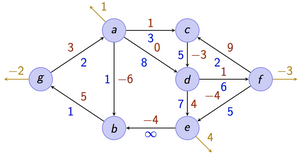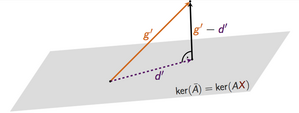# Basic Information

Lecturer: Andreas Karrenbauer Tuesday + Thursday, 14:00 - 16:00, E1.3 HS003; first lecture on Apr 20 Bojana Kodric Sören Bund-Becker, Davis Issac, Pavel Kolev Mondays 10-12, E1.4 024, SörenWednesdays 12-14, E1.4 024, DavisFridays 12-14, E1.4 024, Pavel 9 Basics in linear algebra, discrete mathematics, calculus, algorithms, and complexity. At Saarland University these topics are covered in the bachelor courses Mathematik für Informatiker 1 & 2, Grundzüge der Theoretischen Informatik, and Grundzüge von Algorithmen und Datenstrukturen. Your final grade will be the best of the final exam and the re-exam. You may bring one A4 cheat sheet (double-sided, in your own handwriting) to the exams.Final Exam: August 18th, 13:15 at Günter-Hotz Lecture HallRe-Exam: September 25th, 13:15

If you want to participate in the course, please register to our mailing list!

# Description

This course provides an introduction to fundamental concepts and algorithmic methods for solving linear and integer linear programs.

Linear optimization is a key subject in theoretical computer science. Moreover, it has many applications in practice. A lot of problems can be formulated as (integer) linear optimization problem. For example, combinatorial problems, such as shortest paths, maximum flows, maximum matchings in graphs, among others have a natural formulation as a linear (integer) optimization problem. In this course you will learn:

• how to optimize a linear function subject to linear constraints
• how to formulate combinatorial problems as (integer) linear optimization problems
• how to solve them

To this end, basic concepts from polyhedral theory will be introduced. The simplex algorithm and the ellipsoid method will be presented. The lecture concludes with exact and approximation algorithms for NP-hard optimization problems. There will be theoretical and practical exercises.# Policies

This is a 9-credit-point core lecture ("Stammvorlesung"). There will be two lectures and one exercise session per week. We will hand out exercises every week (usually worth 40 points) and each student should score at least 50% in the first half of the course (first 6 exercise sheets) and 50% in the second half in order to be allowed to take the exam.

Students will also have the chance to gather points by contributing to a wiki. Bonus points will be awarded for contributions to the wiki, i.e., for

• correction of typos
• fixing flaws
• filling gaps

The number of awarded bonus points will be determined in the following way: 10 points per 1k characters, further multiplied with a quality factor between 0 and 1.

# Lectures

The slides of all lectures condensed in a handout can be found here.

DateTopicReferenceHomeworkNotes
Apr 20OverviewSheet 0, Solution 0Slides
Apr 25Integer ProgrammingChapter 6 in [W]; Chapter 11.8 in [BT]Sheet 1, Solution 1Slides
Apr 27Branch and BoundChapter 7 in [W] ; Chapter 11.2 in [BT]Slides
May 2DualityChapter 4.7 in [BT]

Solution 2

Slides
May 4Farkas' Lemma, Strong DualityChapters 4.6 and 4.3 in [BT]Slides
May 9Fourier-Motzkin-EliminationChapter 2.8 in [BT]

Solution 3

Bonus sheet

Slides
May 11Facets, Vertices, Extreme Points, Basic Feasible SolutionsChapter 2.2 in [BT]Slides
May 16Optimality of Extreme Points, Standard FormChapters 2.6 and 2.3 in [BT]

Solution 4

Slides
May 18Degeneracy, Basic (Feasible) Directions, Reduced CostsChapters 2.4 and 3.1 in [BT]Slides
May 23Optimal Bases, Development of the Simplex MethodChapters 3.1 and 3.2 in [BT]

Solution 5

Slides
May 25Public HolidayBonus sheet
May 30Simplex - The full tableau implementationChapter 3.3 in [BT]

Solution 6

Slides
Jun 1Introduction to the Ellipsoid Method - Volume of PolyhedraChapter 8 in [BT]Slides
Jun 6The Ellipsoid MethodChapter 8 in [BT]

Solution 7

Slides
Jun 8The Ellipsoid MethodChapter 8 in [BT]Slides
Jun 13Introduction to the Interior Point MethodChapter 9 in [BT]

Solution 8

Slides
Jun 15Public Holiday
Jun 20The Interior Point MethodChapter 9 in [BT]

Sheet 9,

Solution 9

Slides
Jun 22Integer Optimization, Generalization to PolyhedraChapter 3.2 in [W]Slides
Jun 27Unimodular Matrices, Total UnimodularityChapters 7.3,7.4 in [BT]

Solution 10

Slides
Jun 29

Total Unimodular Matrices, Network Flows

Chapters 7.3,7.4 in [BT]; Chapters 3.2, 3.3 in [W]Slides
Jul 4Network FlowsChapters 7.3,7.4 in [BT]; Chapter 3.3 in [W]

Solution 11

Slides
Jul 6Network FlowsChapters 7.3,7.4 in [BT] ; Chapter 3.3 in [W]Slides
Jul 11Max-Flow Min-CutChapter 8.2 in [MG]

Solution 12

Slides
Jul 13Bipartite MatchingChapter 8.2 in [MG]Slides

Jul 18

Hall's Theorem, Matching PolytopesChapter 8.2 in [MG]Test ExamSlides
Jul 20Matching PolytopesChapter 8.2 in [MG]Slides
Jul 25Approximation AlgorithmsChapter 25 in [S]Slides
Jul 27Max-Cut and SDP relaxationsSlides

# Literature

Good textbook on the topic include: